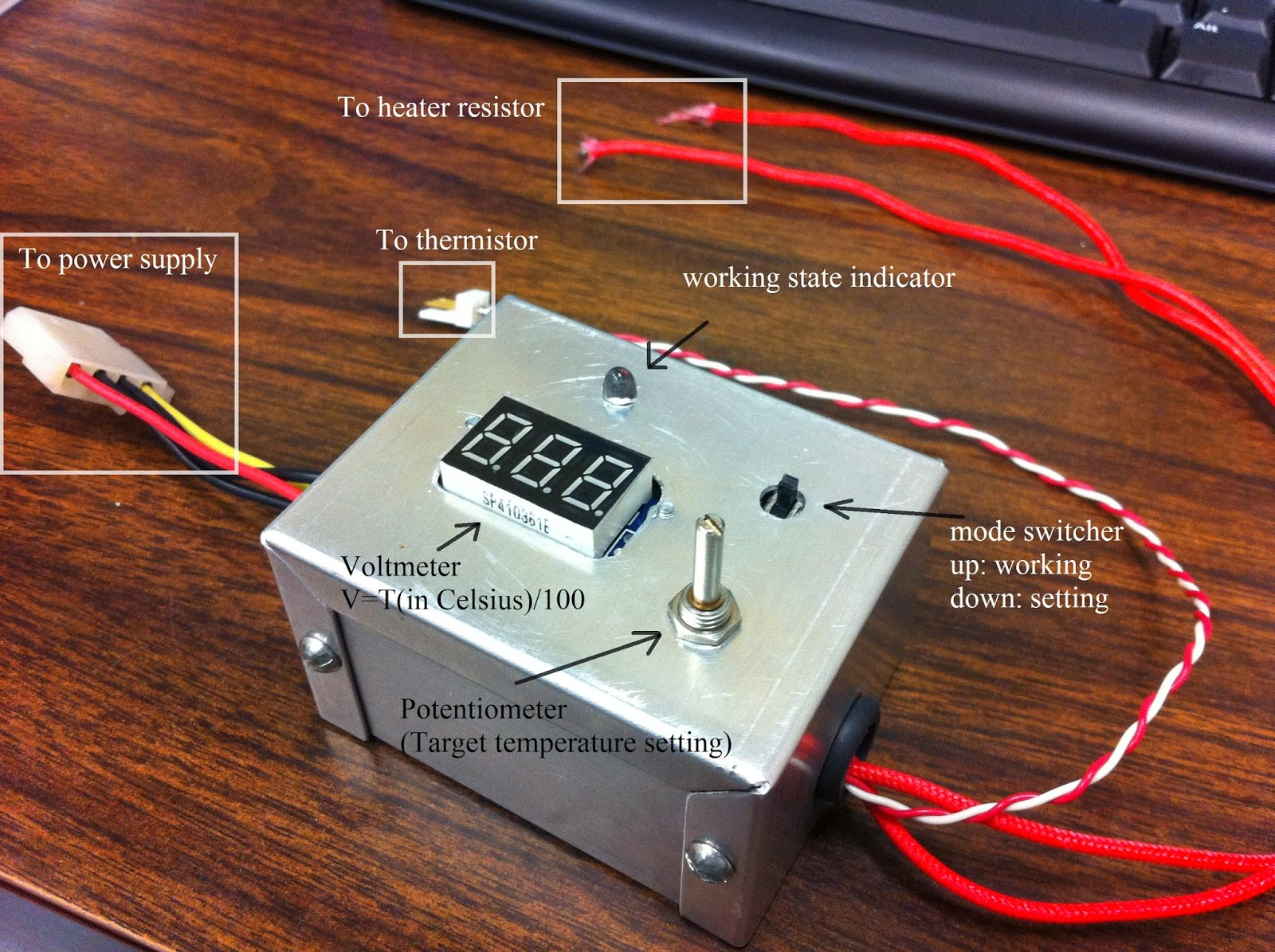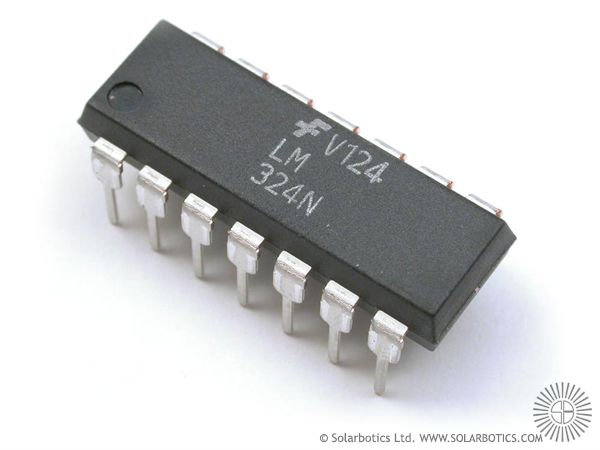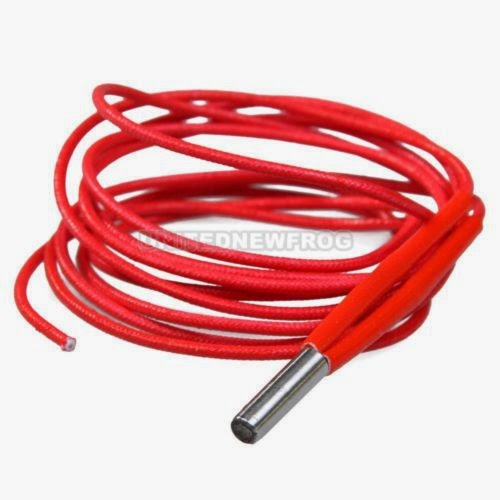## Saturday, March 22, 2014

### A simple temperature control system for 3D printer

[Note: if you are using smartphone or portable device to browser this post, some math formula might not appear properly. To see the math in correct form, scroll down to the bottom and click "View web version"]

After finishing the mini CNC laser engraver, the next thing in my mind is 3D printer. One of the most important part of a 3D printer is the plastic filament extruder, composed by a cold end constantly supplying plastic filament, and a hot end melting the plastic and feeding the liquid plastic through a small nozzle.

For PLA or ABS plastic filaments (most common materials used in home-made 3D printers), the hot end needs to be at a temperature of about 200C (or 180C for PLA, 220C for ABS). It is important to keep this temperature (roughly) constant, so is the plastic melting speed and then a controllable plastic feeding rate.

Therefore two components are essential: a heater, and a temperature sensor. The heater heats up the hot end nozzle, and the sensor monitors the temperature. The heater is on when the nozzle temperature is too low, and is switched off when the nozzle is too hot.

I plan to build a home-made 3D printer controlled by my Raspberry Pi, which, unfortunately, does not have any analog data acquisition pin. Therefore I decide to build a stand-alone temperature control system.

The idea is very simple. I use a power resistor as the heater and a thermistor as the temperature sensor. The system contains an LM324 quad op-amps chip. One op-amp is used as a comparator to compare the thermistor resistance with a nominal resistance and output LOW or HIGH as the comparison result. The other three op-amps inside the LM324 are used to perform some linear transformation and output a voltage that is proportional to the thermistor temperature. This voltage is applied to a 0-30V voltmeter so one can read the temperature. A N-Channel MOSFET transistor is used to control on/off of the heater.

This is the controllerThe finished controller box. About 8cm by 5cm by 4cm

Here is the circuit diagram and some key parts used in the system.Schematics of the temperature control systemLM324 is 14-pin integrated circuit (IC) containing four identical low-profile low-power operational amplifiers (op-amp). The op-amps have a bandwidth of 1MHz, and can be powered by a single power supply with a wide range 3-32V. It is one of the most widely used and lowest cost op-amp ICs. The datasheet can be found here[Note: there is nothing too special about this chip except its compact size. It can be certainly replaced by essentially any other type of op-amps. For the task carried by pin 1, 2 and 3 of LM324 shown in the schematics above, a comparator, instead of an op-amp, is more suitable than LM324.]12V 40W ceramic cartridge heater resistor I used. It is the R_hot in the schematics. They are sold on ebay an other place at a price of about $2/each. For example, here100k ohm NTC 3950 1% thermistor with glass enclosure. It is the R_T in the schematics. They have a wide temperature range from -50C to 260C. Perfect for 3D print (200C). The They cost about $1/each. E.g., here0-30V digital voltmeter. The red lead is for power, black lead for ground, and the blue lead goes to the voltage to be measured. They are about $1.5 each (like this one). Make sure the meter has three wires. There are meters with only two wires (no blue wire) which are not suitable here.IRFP150 N Channel MOSFET transistor. About$1.5/each (like this one). From left to right are the pins of G, D and S. Data sheet can be downloaded here. It can be replaced by similar high power N MOSFET transistors like IRFP140 or IRFZ24

### Choice of the resistors

The choice for the resistors R0, R1, R2, R3 and R4 are crucial. They need to be carefully calculated so that the meter can show correct temperature.

I choose:
R0=600 ohm
R1=10k ohm
R2=3.9k ohm
R3=6.19k ohm
R4=2.4k ohm
R5=10k ohm

I will show how these numbers come out.

### About the thermistor

The NTC thermistor I use has a beta value of 3950 and resistance of 100k ohm at room temperature (~25C, or rounghly 300Kelvin).

NTC stands for Negative Temperature Coefficient, meaning that the resistance of the thermistor decreases as the temperature increases.

The Steinhart Hart equation for NTC thermistor is
$$\frac{1}{T}=\frac{1}{T_0}+\frac{1}{B}ln\left(\frac{R}{R_0}\right)$$
where $$T_0$$ is room temperature in Kelvin (~300), B=3950 is the coefficient, $$R_0$$ is the resistance of the thermistor in ohm at room temperature, i.e., 100000. Then given temperature $$T$$ in Kelvin we can calculate the resistance of the thermistor, or given a resistance we can calculate the temperature.

The S-H equation is a highly non-linear equation. It is, however, possible to use a simple linear function to approximate the S-H equation within some narrow temperature range.

### How the temperature meter works

The system has two modes: normal working mode, and temperature setting mode. The switch SW controls which mode is selected. When SW is at 1 (default), the system is at normal working mode. When SW is at 2 (temporal), the system allows you to set the desired temperature.

The LM324 has four op-amps.Pin 4 and 11 are the Vcc and Vee. I use a single 12V power supply to power the whole system so pin 4 is connected to +12V and 11 is connected to ground. Pin [1,2,3], [5,6,7],[8,9,10] and [12,13,14] are the four op-amps.

Op-amp [1,2,3] is used as a voltage comparator. I will discuss this later.
(It is usually not recommended to use an op-amp as a comparator. But it does work here because LM324 accepts true differential input signals into its inverting and non-inverting inputs).

Op-amp [5,6,7] behaves simply as a voltage follower. Pin 6 and 7 repeats whatever voltage Pin 5 has without draining current from pin 5. In normal working mode (SW at 1), the voltage of Pin 5 is the voltage between the thermistor and R0. We define this voltage, the voltage of the junction of thermistor R_T and R0, as $$Vin$$.

With R0, there is
$$Vin=\frac{R0}{R0+R_T}12 V=Pin5=Pin6=Pin7$$

Then let's move to resistor R1 and R2. Clearly, the voltage at pin 10 is
$$\frac{R2}{R1+R2}\cdot12+\frac{R1}{R1+R2}\cdot Vin=\frac{R1}{R1+R2}\left(\frac{R2}{R1}\cdot12+Vin\right)$$

Op-amp [8, 9, 10] is another voltage follower. Hence Voltage of Pin 8 = Pin 9 = Pin 10.

Resistor R3 and R4 form a voltage divider. The voltage between R3 and R4 is
$$\frac{R4}{R3+R4}\cdot Pin9=\frac{R4}{R3+R4}\frac{R1}{R1+R2}\left(\frac{R2}{R1}\cdot 12+Vin\right)$$

Op-amp [12,13,14] is another follower. There is Pin 14=Pin 13 = Pin 12= voltage between R3 and R4.

We define the voltage at Pin 14 is $$Vout$$. Hence
$$Vout=\frac{R4}{R3+R4}\frac{R1}{R1+R2}\left(\frac{R2}{R1}\cdot 12 + Vin \right)$$

Now plug into the numbers: R1=10K ohm, R2=3.9k ohm, R3=6.19k ohm, R4=2.4k ohm, we get
$$Vout=0.279\times 0.719\times (0.39\times 12+Vin)=0.2(4.7+Vin)$$

All the three op-amps do is to perform this linear scaling.

Here is the magic. I claim that, with R0=600ohm and $$Vin=600/(600+R_T)\times12$$V, there is
$$Vout=T/100$$
where T is the temperature of the thermistor in Celsius. This equation is true for a quite wide temperature range 120C-260C.

The figure below is the proof. It is shown that with R0=600ohm, $$Vin$$ is quite linear in the range of 120C to 260C. Therefore we can perform some linear transformation to map $$Vin$$ linearly to T/100.Top left: thermistor resistance at different temperature. Top right: same as top left but with axes switched. Lower left: with R0=600ohm, Vin as function of temperature from 90C to 280C. Lower right: Vout as function of Temperature (blue) and T/100 (red, T in Celsius)

To map Vin to T/100, we perform linear regression between Vin and T/100, and obtain
$$\frac{T}{100}=0.2Vin+0.94=0.2(Vin+4.7)$$

Recall that
$$Vout=\frac{R4}{R3+R4}\frac{R1}{R1+R2}\left(\frac{R2}{R1}\cdot 12 + Vin \right)$$
If we want to have $$T/100=Vout$$, there must be
$$\frac{R2}{R1}=4.7/12=0.39$$
and
$$\frac{R4}{R3+R4}\frac{R1}{R1+R2}=0.2$$

Since $$R2/R1=0.39$$ gives $$R1/(R1+R2)=0.719$$, the second requirement is reduced to
$$\frac{R4}{R3+R4}=0.2/0.719=0.278$$

Therefore [R1=10K ohm, R2=3.9k ohm, R3=6.19k ohm, R4=2.4k ohm] is an obvious solution (not the only one though).

Here is a list for key parameters at different temperatures

T(C)    R_T(k ohm)     Vin (V)      Vout (V)

120          4.0594          1.5453        1.2491
140          2.4950          2.3263        1.4053
160          1.6041          3.2667        1.5933
180          1.0723          4.3055        1.8011
200          0.7416          5.3666        2.0133
220          0.5285          6.3801        2.2160
240          0.3867          7.2969        2.3994
260          0.2897          8.0928        2.5586

Note that the $$Vout=T/100$$ relation is preserved very good within the range of 140C to 240C. Detailed calculation shows that the averaged errors from 140C to 240C is less than 0.5%. Very good!

We feed this Vout to a voltmeter so the voltmeter reading is T/100.

### How the temperature control works

The temperature control is done by the op-amp [1,2,3]. Toggle the SW to position 2. The meter is reading a temperature assuming the potentiometer R is R_T and R0. We set the potentiometer R so that the meter reads the desired value, say 2V (corresponding to 200C). Then toggle the SW back to position 1.

The voltage of Pin 3 is then a constant. The voltage of Pin 2 equals to Pin 3 when and only when the temperature is 200C.

Below 200C, R_T is too large. So the Pin 2<Pin 3. The op-amp is trying to amplify the voltage difference between its non-inverting (pin 3) and inverting pins (pin 2) by hundreds of thousands times. But it can't output any voltage above the power supply voltage-1.5V Therefore it outputs 12-1.5=10.5V through pin 1. The Gate-Source voltage of the MOSFET transistor is then large enough and the D-S of the MOSFET become conductive. The heater is then switched on.

When the temperature hits above 200C, R_T is too small. So the Pin 2>Pin 3. The op-amp outputs zero at Pin 1. The MOSFET is switched off and so is the heater.

After the temperature drops below 200C again, the cycle repeats.

So it is a negative feedback system. The temperature is maintained around 200C.

Note that when Pin 1 outputs 10.5V, the LED is also switched on to indicate that the heater is working.

### Some remark

The system accomplishes two major tasks: displaying the temperature correctly and maintaining a constant temperature at the location of thermistor.

The first task is accomplished by using three op-amps. The limitation is that the system can only display temperature correctly within a certain range. Here, in our case, is 120C-260C. Below 120C or above 260C the system behaves very poor.

Mathematically it is guaranteed that the op-amps idea will definitely work essentially for any system, but within some limited range. This is because in most times the Taylor-expansion of any smooth function $$f(x)=f(x_0)+(x-x_0)f'(x_0)+...$$ always has a linear term $$(x-x_0)f'(x_0)$$. One can always use a linear function to approximate the real function. On the other hand, this approximation only works within a narrow range.

I feel very lucky that the linear range in my case is so wide (120C-260C).

To properly display the temperature in wider range, say, 0C to 300C, it is necessary to use some micro controller to perform the complicated T-R conversion calculation. But micro controller is is far more complicated and expensive than LM324.

My idea can be easily extended to other system. For example, a constant temperature water-filling container (what does it for? Say, 45C Baby milk warmer). One need to linearize the system around 45C and find proper R0-R4.

A note is that LM324 cannot output voltage below 0 or above power supply voltage-1.5V. So every step of the linearization shouldn't involve any voltage beyond this range.